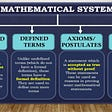# Demand Forecasting in Supply Chain Management-A time-series approach (2/2)

## Cleaning and preprocessing

`df_features.head()`
`df_features.isna().sum()`
`df_features.CPI.plot();`
`df_features.Unemployment.plot();`
`for i in range(1,46):    df_features[df_features.Store==i] =    df_features[df_features.Store == i].interpolate()`
`df_features[df_features.columns[4:9]] = df_features[df_features.columns[4:9]].fillna(0)`
`df_all_1 = df_features.merge(df_sales, 'right', on = ['Date', 'Store', 'IsHoliday'])df_all = df_all_1.merge(df_stores, 'left', on = 'Store')df_all = df_all.sort_values('Date')df_all.reset_index(inplace = True)`
`df_all.replace({'IsHoliday':{True:1, False:0}}, inplace=True)df_all.replace({'Type':{'A':3, 'B':2, 'C':1}}, inplace=True)`

## EDA

`df_by_date = df_all.groupby('Date',as_index=False).agg({'Temperature': 'mean', 'Fuel_Price': 'mean', 'CPI': 'mean', 'Unemployment': 'mean', 'Weekly_Sales': 'sum', 'IsHoliday': 'mean'})df_by_date.Date = pd.to_datetime(df_by_date.Date, errors='coerce')df_by_date.set_index('Date', inplace=True)`
`df_by_date_new = df_by_date.resample('W').mean().fillna(method='bfill')`
`from statsmodels.tsa.seasonal import seasonal_decomposemulti_plot = seasonal_decompose(df_by_date_new['Weekly_Sales'], model = 'add', extrapolate_trend='freq')`
`multi_plot.observed.plot(title = 'weekly sales')`
`multi_plot.trend.plot(title = 'trend')`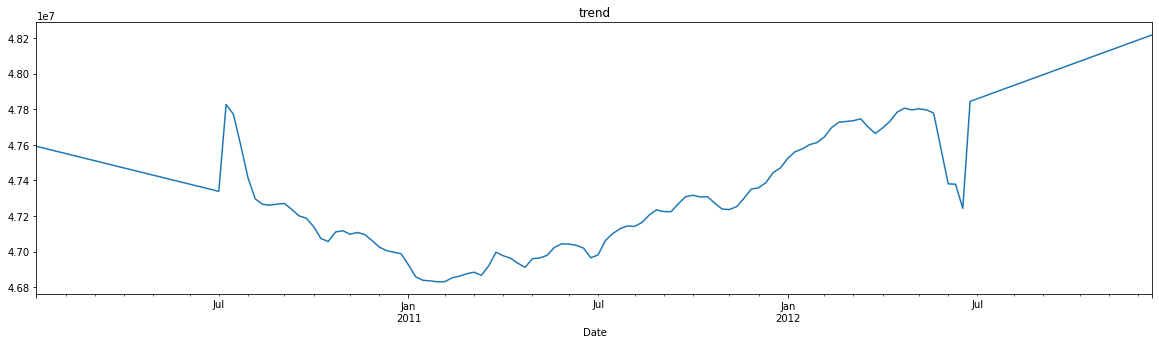A fairly flat trend (note that y-axis limits are close to each other)
`multi_plot.seasonal.plot(title = 'seasonal')`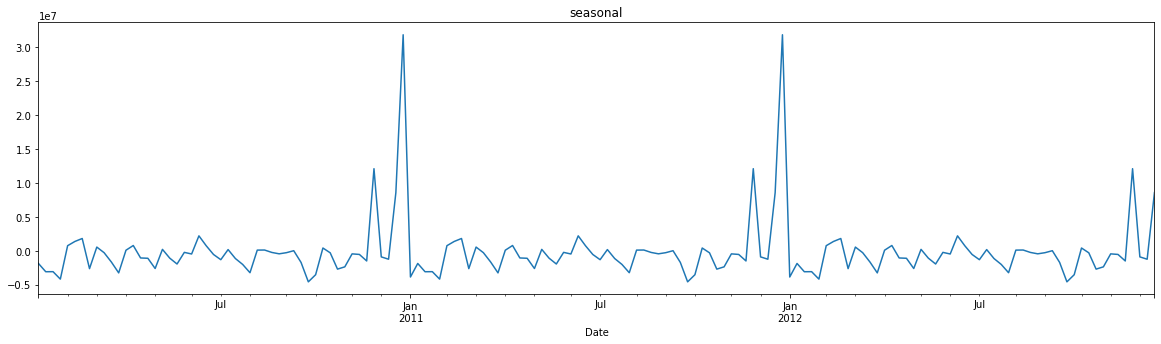Strong seasonality that tends to kick in during the Nov-Dec period
`multi_plot.resid.plot(title = 'residual')`
`sns.heatmap(df_by_date_new.corr('spearman'), annot = True)`
• strong +ve correlation b/w Fuel_Price and CPI
• strong -ve correlations b/w Unemployment and Fuel_Price and Unemployment and CPI
• surprisingly, the unemployment rate doesn’t really seem to affect the weekly sales (directly at least) suggesting that the stores might be overstaffed.
`sns.boxplot(data = df_by_date, x = 'IsHoliday', y = 'Weekly_Sales');`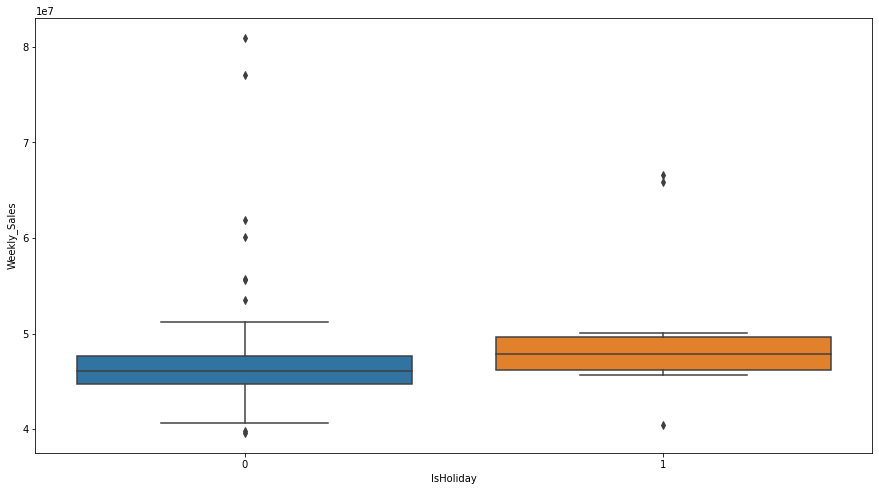holiday weeks don’t necessarily mean that the weekly sales go up but it is often the case
`df_by_store = df_all.groupby('Store').agg({'Weekly_Sales': 'sum',                                           'Type': 'max'})sns.boxplot(data = df_by_store, x = 'Type', y = 'Weekly_Sales')`
`monthly_sales = df_all.groupby(df_all.Date.dt.month).agg({'Weekly_Sales':'sum'})sns.barplot(x=monthly_sales.index, y=monthly_sales.Weekly_Sales);`
`df_by_dept = df_all.groupby('Dept').agg({'Weekly_Sales':'sum'})df_by_dept.sort_values(by = 'Weekly_Sales', ascending = False, inplace = True)`

## Forecasting using the Holt-Winters Model

`from statsmodels.tsa.holtwinters import ExponentialSmoothing as esfit_model = es(df_by_date_new['Weekly_Sales'[:120], trend = 'add',                 seasonal = 'add', seasonal_periods = 52).fit()prediction = fit_model.forecast(34) `
`plt.plot(df_by_date_new.index[120:], prediction, 'predicted')plt.plot(df_by_date_new.index[120:], df_by_date_new.Weekly_Sales[120:], 'actual')plt.legend();`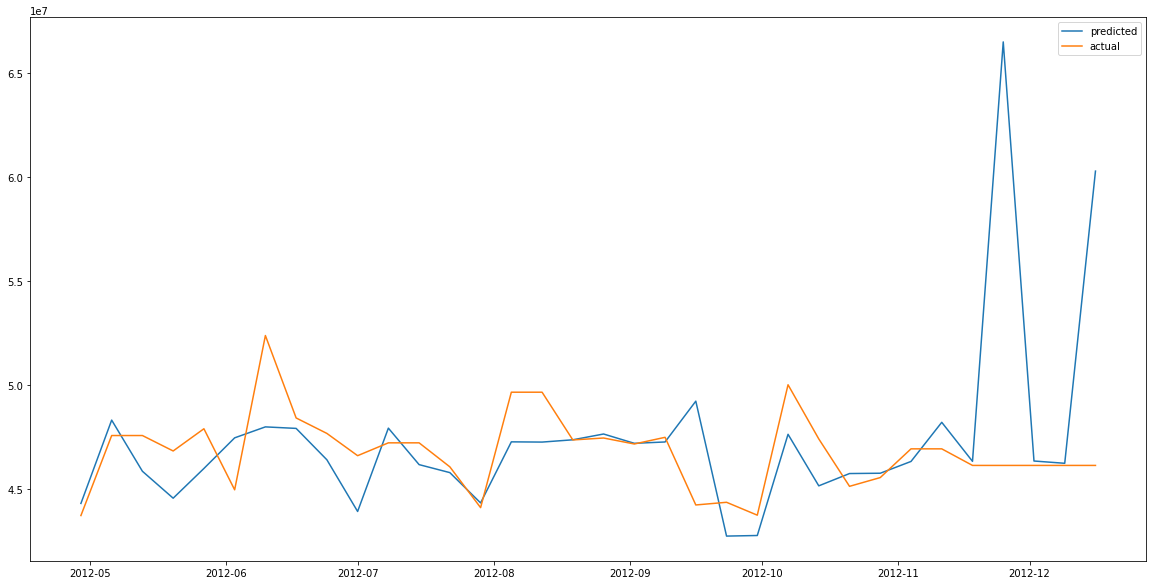Our model follows the general trend till the seasonality component kicks in during the Christmas period. Note that a similar peak was observed in all the other years as well during Christmas time.
`def mean_absolute_percentage_error(y_true, y_pred):     return np.mean(np.abs((y_true - y_pred) / y_true)) * 100print("Mean Absolute Percentage Error = {a}%".format(a=mean_absolute_percentage_error(df_by_date_new.Weekly_Sales[120:],prediction)))`
`fit_model = es(df_by_date_new['Weekly_Sales'][:-2],               trend = 'add',seasonal='add',               seasonal_periods=52).fit()preds_2013 = fit_model.forecast(56)plt.plot(df_by_date_new.index, df_by_date_new.Weekly_Sales)plt.plot(preds_2013, '--')plt.legend(['2010-2012 actual', '2013 forecast'])`

--

--

--

## More from Sigma

Love podcasts or audiobooks? Learn on the go with our new app.

## How Effective Analytics Brings Clarity in the Age of Pandemics## How to Create a Seaborn Palette That Highlights Maximum Value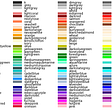## Data analytics and data integration are at the core of an omnichannel business## A Deep Dive into African Data Science on Kaggle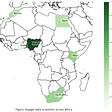## How Do YOU Become A Good Data Scientist?## Practical_Exam_Work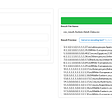## Python3: Mutable, Immutable… everything is object!## Short-Circuiting (&& ||) and Nullish Coalescing Operator (??)## MATHEMATICAL SYSTEM